Balbharati Maharashtra State Board Std 12 Commerce Statistics Part 1 Digest Pdf Chapter 8 Differential Equation and Applications Ex 8.5 Questions and Answers.

## Maharashtra State Board 12th Commerce Maths Solutions Chapter 8 Differential Equation and Applications Ex 8.5

Solve the following differential equations.

Question 1.
$$\frac{d y}{d x}+y=e^{-x}$$
Solution:
$$\frac{d y}{d x}+y=e^{-x}$$ …….(1)
This is the linear differential equation of the form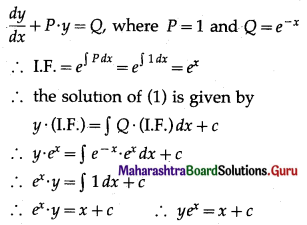This is the general solution.

Question 2.
$$\frac{d y}{d x}$$ + y = 3
Solution:
$$\frac{d y}{d x}$$ + y = 3
This is the linear differential equation of the form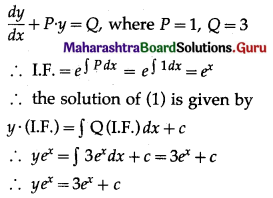This is the general solution.Question 3.
x$$\frac{d y}{d x}$$ + 2y = x2 . log x.
Solution:
x$$\frac{d y}{d x}$$ + 2y = x2 . log x
∴ $$\frac{d y}{d x}+\left(\frac{2}{x}\right) \cdot y=x \cdot \log x$$ …….(1)
This is the linear differential equation of the form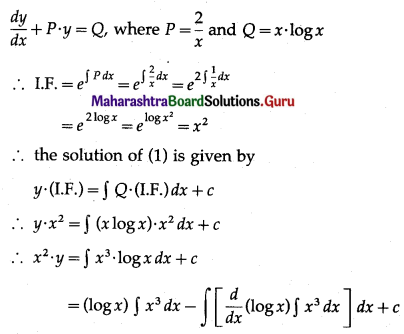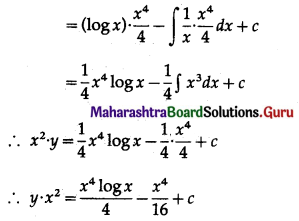This is the general solution.

Question 4.
(x + y)$$\frac{d y}{d x}$$ = 1
Solution:
(x + y) $$\frac{d y}{d x}$$ = 1
∴ $$\frac{d x}{d y}$$ = x + y
∴ $$\frac{d x}{d y}$$ – x = y
∴ $$\frac{d x}{d y}$$ + (-1) x = y ……(1)
This is the linear differential equation of the form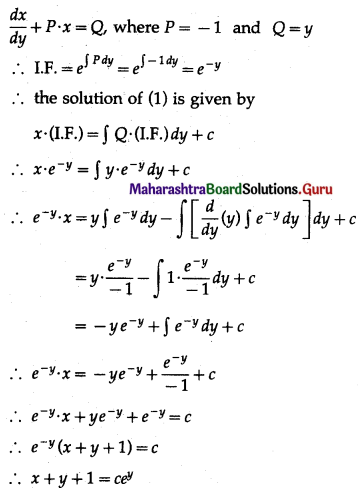This is the general solution.

Question 5.
y dx + (x – y2) dy = 0
Solution:
y dx + (x – y2) dy = 0
∴ y dx = -(x – y2) dy
∴ $$\frac{d x}{d y}=-\frac{\left(x-y^{2}\right)}{y}=-\frac{x}{y}+y$$
∴ $$\frac{d x}{d y}+\left(\frac{1}{y}\right) \cdot x=y$$ ……(1)
This is the linear differential equation of the form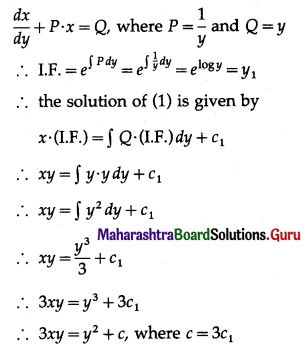This is the general solution.Question 6.
$$\frac{d y}{d x}$$ + 2xy = x
Solution:
$$\frac{d y}{d x}$$ + 2xy = x ………(1)
This is the linear differential equation of the form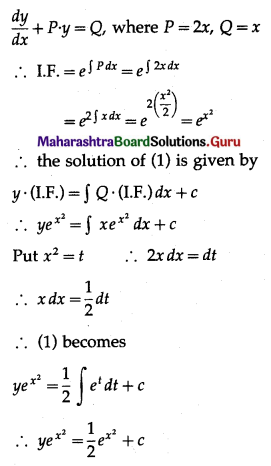This is the general solution.

Question 7.
(x + a) $$\frac{d y}{d x}$$ = -y + a
Solution:
(x + a) $$\frac{d y}{d x}$$ + y = a
∴ $$\frac{d y}{d x}+\left(\frac{1}{x+a}\right) y=\frac{a}{x+a}$$ ……..(1)
This is the linear differential equation of the form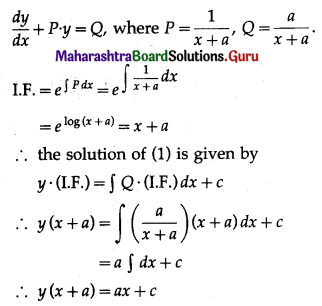This is the general solution.Question 8.
dy + (2y) dx = 8 dx
Solution:
dy + (2y) dx = 8 dx
∴ $$\frac{d y}{d x}$$ + 2y = 8 …….(1)
This is the linear differential equation of the form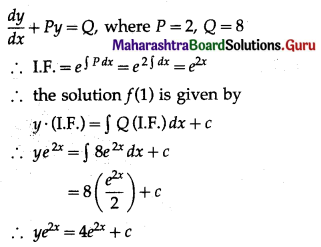This is the general solution.